Detailed Acoustic Analysis of a Home Studio

There are many factors determine the acoustical properties of a room, such as the shape, the structure, dimensions, surface materials and also the furnishing. To get an overall picture about the acoustical properties need to do the theoretical calculations and measurements as well. The modern software that I used, the REW V5 is able to precisely measure the acoustic properties of a room. Also it is also able to analyze and show the frequency responses and the RT60 values from a given impulse response file.

This paper will analyse and compare the theoretical calculations, such as room modes and reverberation time, and the practical measurements of the given room. It will be interesting to see the differences between the calculated and measured values.

Overview of the Room

The analyzed room’s shape is roughly rectangular. Thus the walls as well as the ceiling and the floor are broadly parallel to each other, thus we assume that these are perfectly parallel. This assumption may create differences in the determination of room modes. All the walls and ceiling are constructed of concrete and and the floor is covered with tiles.

The room dimensions:

Length: 4.8m
Width: 3.4m
Height: 3.1m

Bolt Ratio

The geometry of the room affects the acoustical performance and the relative proportion of length, width and height determine the acoustic suitability. There are early guesses and statistical analyses of room proportion that give nearly even modal distribution. Dr. Richard H. Bolt American physics professor developed a diagram - Figure 1 - of room proportion that gives smooth room characteristics at low frequencies in small rectangular rooms. The following researchers Sepmeyer, Louden, Volkmann and Boner shared different ratio suggestions. Most of them fall on or close the Bolt area as we see on Figure 2. (Everest & Pohlmann, 2009, p. 247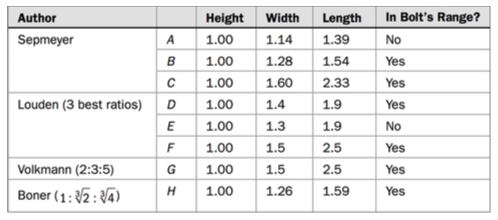Figure 1. Rectangular room dimensions ratio suggestions by different researchers

This parameters gives the following ratio: 1:1.1:1.54 which doesn’t match with the ideal ratios, regarding to Boner suggested ‘perfect’ small room ratio (1:1.26:1.59). (Boner, 1942)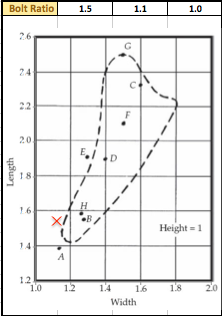Figure 2. Bolt ratio diagram - the red "X" shows the actual room's ratio which is out of the field.

The room has tile floor which is approximately 20% covered with a thin carpet. The window covers the approximately 30% of the front wall, but 70% of the wall covered with thick curtain in front. The back wall is not straight therefore the room shape is not symmetrical, thus it might cause some deviation between the calculated and the measured numbers.

Measuring Equipment

To capture the impulse responses of the rooms I used Behringer ECM8000 calibration microphone, Apogee Duet 2 professional audio interface and the free REW V5 measuring software.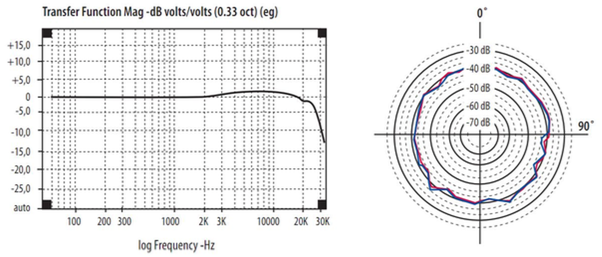Figure 3. Frequency response of Behringer ECM800 calibration microphone

Room Modes

Room modes are a collection of resonances in a room occurred by a sound source such as a loudspeaker. There are three room modes differentiated depending on the type of the reflection. Room modes occur at certain frequencies, which are directly related to the room’s dimensions. Thus to identify these frequencies we need to know the dimensions of the room and the speed of the sound. (Everest & Pohlmann, 2009, p. 228) These resonances occur between the lowest fundamental frequency (first axial mode) and the cutoff frequency (fc) of the room. This will be discussed in details later.

The speed of sound varies on different temperatures and let's say in our case the room’s temperature is stable 20 degree Celsius. Using the calculator on “sengpielaudio.com” we get the exact speed, which is rounded to two decimal places. (Sengpiel)

c20 = 343.22m/s

Axial modes

The axial modes are established between two reflective parallel and opposite walls. We can calculate the first axial modes of the three dimensions f1 or with other words the fundamental frequencies using the following formula, where c = the speed of sound in meters per second and L = the distance in meters between the two walls.

f1 = c/2L

These fundamental frequencies are accompanied by a series of modes each of which also exhibits resonance. These modes are the exact multiples of the fundamental frequency. (Everest & Pohlmann, 2009, p. 228)

f2 =2f1; f3 =3f1; etc.

Applying these equations on the given room first we get the three fundamental frequencies or other words the three first axial modes and multiplying the result we get the second, third, fourth and etc. resonances. In practice those sounds on an instrument that close to these frequencies would sound louder than the other ones.

f-lenght = 35.75 Hz; f-widht = 49.45Hz; f-height = 55.35Hz

As we see on Figure 4. the distribution of the axial modes are not uniform. Some of the modes are located in more densely while other ones are less densely. The uneven distribution might causes peaks and dips on the frequency response. To get an accurate conclusion we need to calculate the tangential and oblique modes then display and summarise these modes in one plot.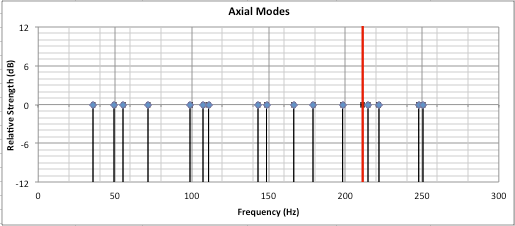Figure 4. The distribution of axial modes

Tangential modes

The tangential modes reflect off four reflective walls, these can be two sets of parallel walls, or two parallel walls and the ceiling and the floor. These modes have about half of the energy of the axial modes. (Wieczorek, 2002) The following equation required to get the frequencies (f) where the tangential modes occur where L, W, H are the dimensions of the room, length, width, height in meter. The p, q, r are integers which indicates the which mode we calculate and the level of the multiplication. For example: (1,0,0) indicates the first axial mode, or (2,2,0) indicates the second tangential mode. (Everest & Pohlmann, 2009, p. 230)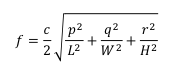Figure 5. Equation for Tangential Modes

Applying this equation we can get the tangential modes of the given room. The first three tangential modes occur at (1,1,0) = 61.16Hz; (1,0,1) = 66.05Hz; (0,1,1) = 74.40Hz. As the tangential modes are less powerful than the axial modes, those are referenced at -3dB on the plot (Figure 6). As we see the distribution of the modes are uneven and at higher frequencies are dense. There are certain modes that might affect the frequency response of the room, such as two or more modes occur at around 120Hz, 180Hz and 200Hz. At these frequencies the modes occur so close almost overlap each other. At some other places the modes are located a longer distance from each other such as around 100Hz and 140Hz. This unevenness might cause dips in the frequency spectrum.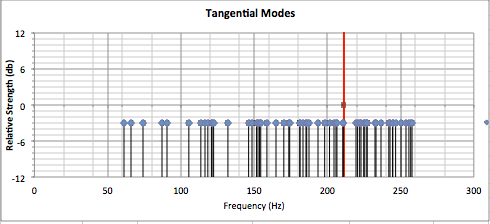Figure 6. The distribution of tangential modes

Oblique Modes

The oblique modes reflect off all the six reflective surfaces including walls, ceiling and the floor. These modes are one quarter as strong as the axial modes and half as strong as the tangential modes. (Wieczorek, 2002) The same equation what we used to get the tangential modes required to get the frequencies (f) where the oblique modes occur, where L, W, H are the dimensions of the room, length, width, height in meter. The p, q, r are integers which indicates the which mode we calculate and the level of the multiplication. (Everest & Pohlmann, 2009, p. 230)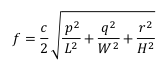Figure 7. Equation for Oblique Modes

Applying the equation in practice it gives us that the first oblique mode occur at (1,1,1) = 82.58Hz As I mentioned the oblique modes are half as strong as tangential modes and one quarter as strong as axial modes, therefore the oblique modes are referenced on -6dB on the plot (Figure 8). As we previously seen the axial and tangential modes distribution uneven and no difference to the oblique modes. The second (2,1,1) oblique mode occurs at 103.30Hz, which is far from the first mode (1,1,1), which occurs at 82.58Hz, this might cause changes on the frequency plot. There are also differences may occur on the frequency spectrum at certain frequencies where the modal density is uneven and outstandingly high.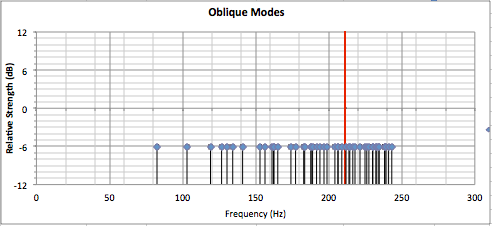Figure 8. The distribution of oblique modes

All Modes

Summarising the axial, tangential and oblique modes in one plot will give a better picture about the problematic areas where the modal density outstandingly high or low. The modal density is increasing with the frequency, we see on Figure 8 and the previous plots (Everest & Pohlmann, 2009, p. 244)

As we see on Figure 6 some of the adjacent modes are overlap or really close to each other. In practice this means that energising one mode will tend to force the adjacent mode or modes into excitation. It is really difficult to determine whether the coincidence of modes leads to a resonant peak or a dip on the frequency response plot. Taken modes separately, each one presents a resonant peak. (Everest & Pohlmann, 2009, p. 239)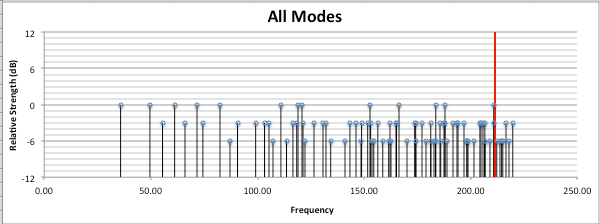Figure 9. The distribution of axial, tangential and oblique modes

The first problematic area is at where the first axial mode (0,1,0) = 49.57Hz and the other fundamental modes meet: 55.48Hz, 61.16Hz, 66.05Hz and 71.67Hz. Since many of them are fundamental (first level) modes, probably it will show a resonant peak or dip on the frequency response graph. The same phenomena might happen at the dense or uneven areas. Now lets move to the measured numbers.

Analysis of the Impulse Responses

Probably some derogation will be between the theoretical and actual values. As we see on Figure 10. the frequency response contains not negligible peaks and dips, especially in the low range. Whereas the room is empty there is nothing dampening the resonant frequencies. Lets say our reference level is at 97dB. There is approximately 15dB peak at around 110Hz, which is probably caused by the meet of one of the axial and tangential modes. Comparing the modes distribution graphs to the frequency response graph (Figure 10. blue line) we can find that the peaks and dips occur really close to the calculated room modes.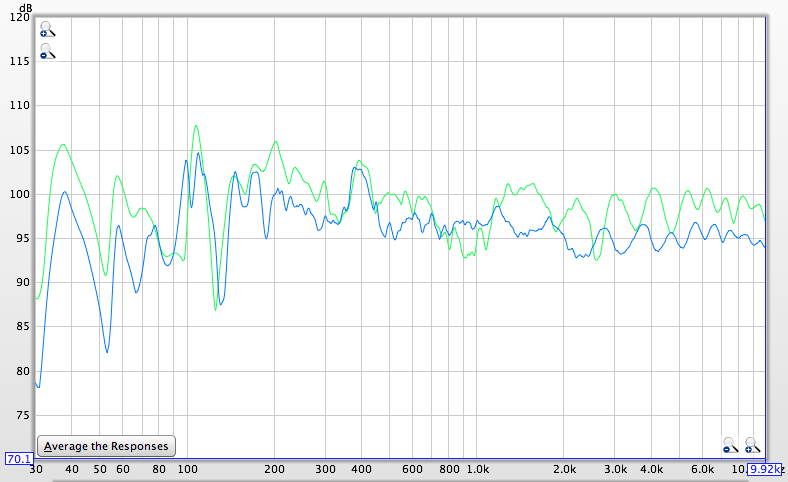Figure 10. Frequency Response (FR) plot of the measured room. The blue line indicates the FR in the empty room.

The waterfall plot shows those frequencies where the resonances occur in the room. Easy to find these frequencies, we just need to locate those long tails. So the waterfall plot clearly confirms the previous assumptions. As we see on Figure 11. there is a huge resonant peak at 108Hz, but we can find many other ones above and below as well such as: 60Hz, 80Hz and 100Hz.Figure 11. Waterfall plot of the empty room.

Conclusion

Based on the previous comparison the theoretical calculations of modal resonances are surprisingly close to the measure frequency response. The large peaks and dips occur on the frequency response, because the room is basically empty and there are no absorption materials, which would be able to absorb the low frequency modal resonances. So basically this is the calculation of the theoretical acoustic properties of the actual room. The calculation of the RT60 values completely unnecessary, because the measured and calculated numbers are always widely different.